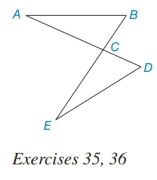Chapter 3.1, Problem 36E### Elementary Geometry for College St...

6th Edition
Daniel C. Alexander + 1 other
ISBN: 9781285195698

#### Solutions

Chapter
Section### Elementary Geometry for College St...

6th Edition
Daniel C. Alexander + 1 other
ISBN: 9781285195698
Textbook Problem
1 views

# In Exercises 33 to 36, the method to be used are SSS, SAS, ASA, and AAS. Given that ∠ A ≅ ∠ E and B C ¯ ≅ D C ¯ , does it follow that Δ A B C ≅ Δ E D C ? Cite the method, if any, used in reaching at this conclusion. (See the figure for Exercise 35 .)To determine

To find:

The method for the given congruency.

Explanation

Given:

The triangles ΔABC and ΔEDC are shown in the figure below,

Figure (1)

From the figure (1),

AE

And,

BD

If two lines bisect, then the vertical angles formed are congruent.

Approach:

Consider the triangles,

ΔABC and ΔEDC

From the given data,

&

### Still sussing out bartleby?

Check out a sample textbook solution.

See a sample solution

#### The Solution to Your Study Problems

Bartleby provides explanations to thousands of textbook problems written by our experts, many with advanced degrees!

Get Started